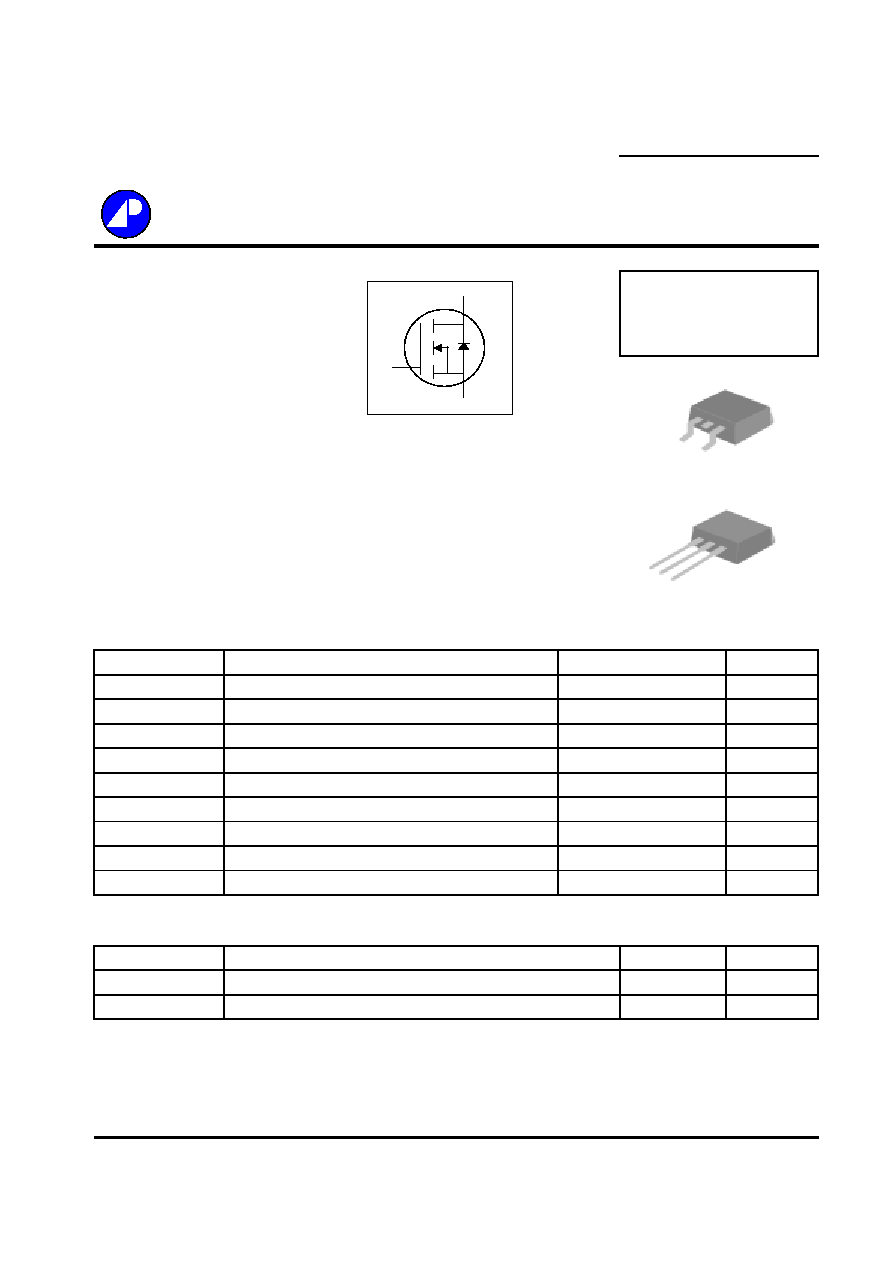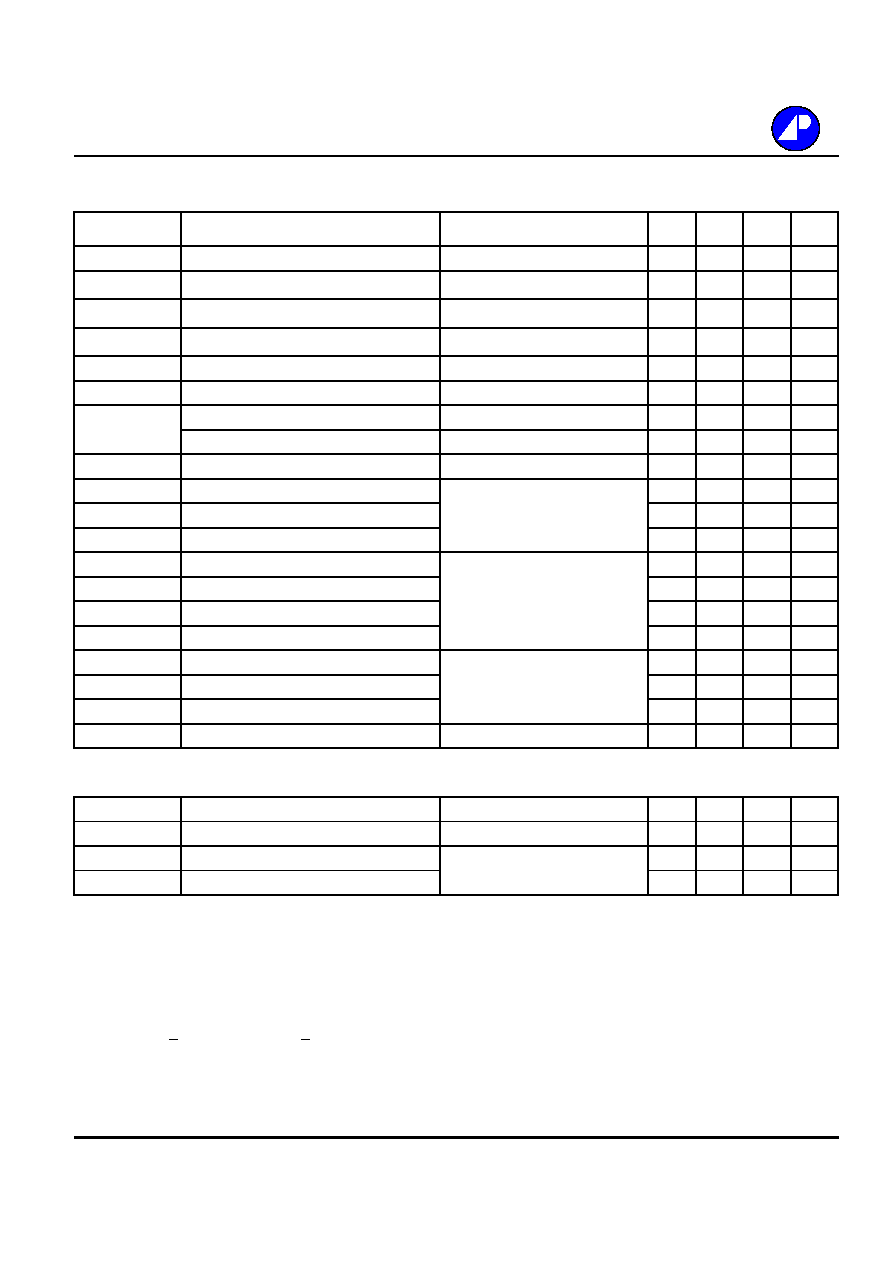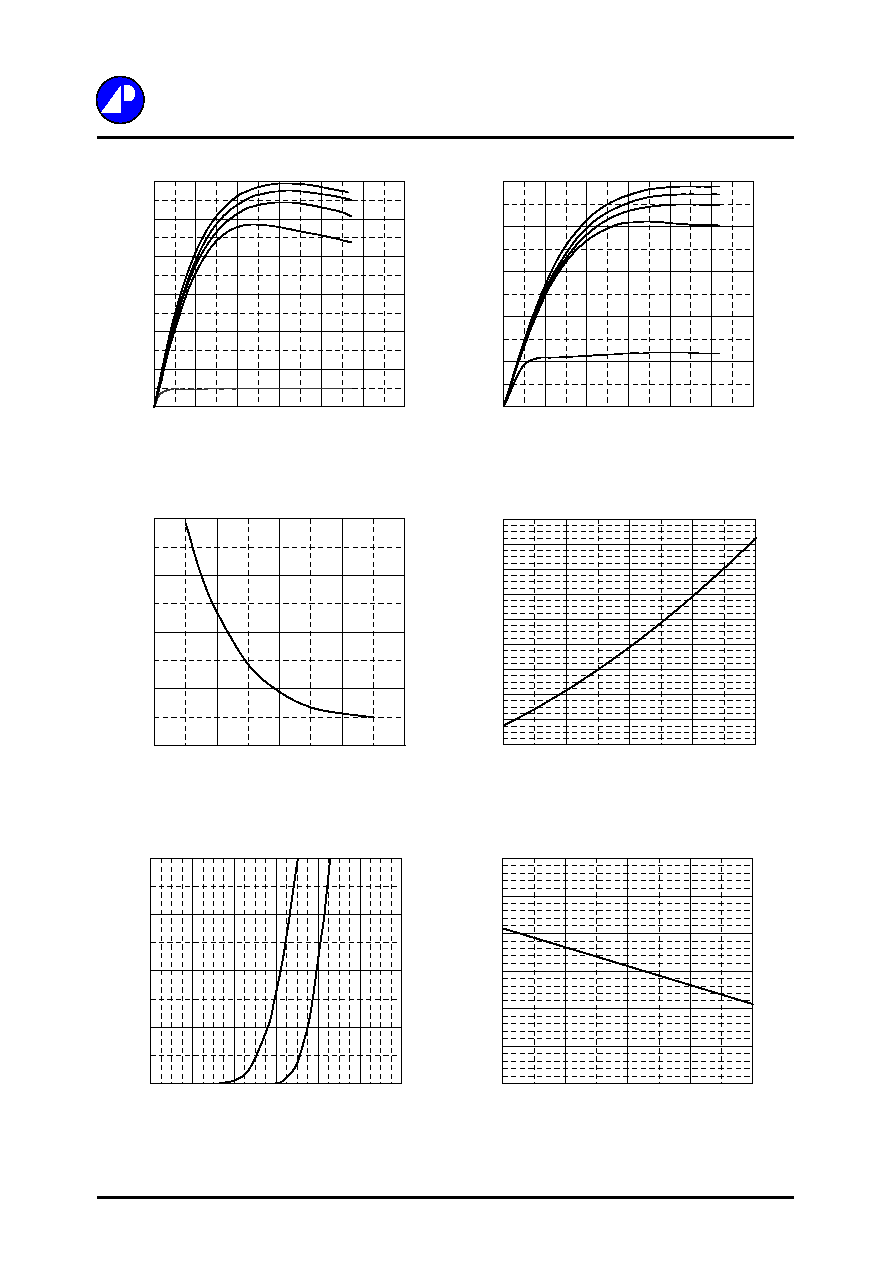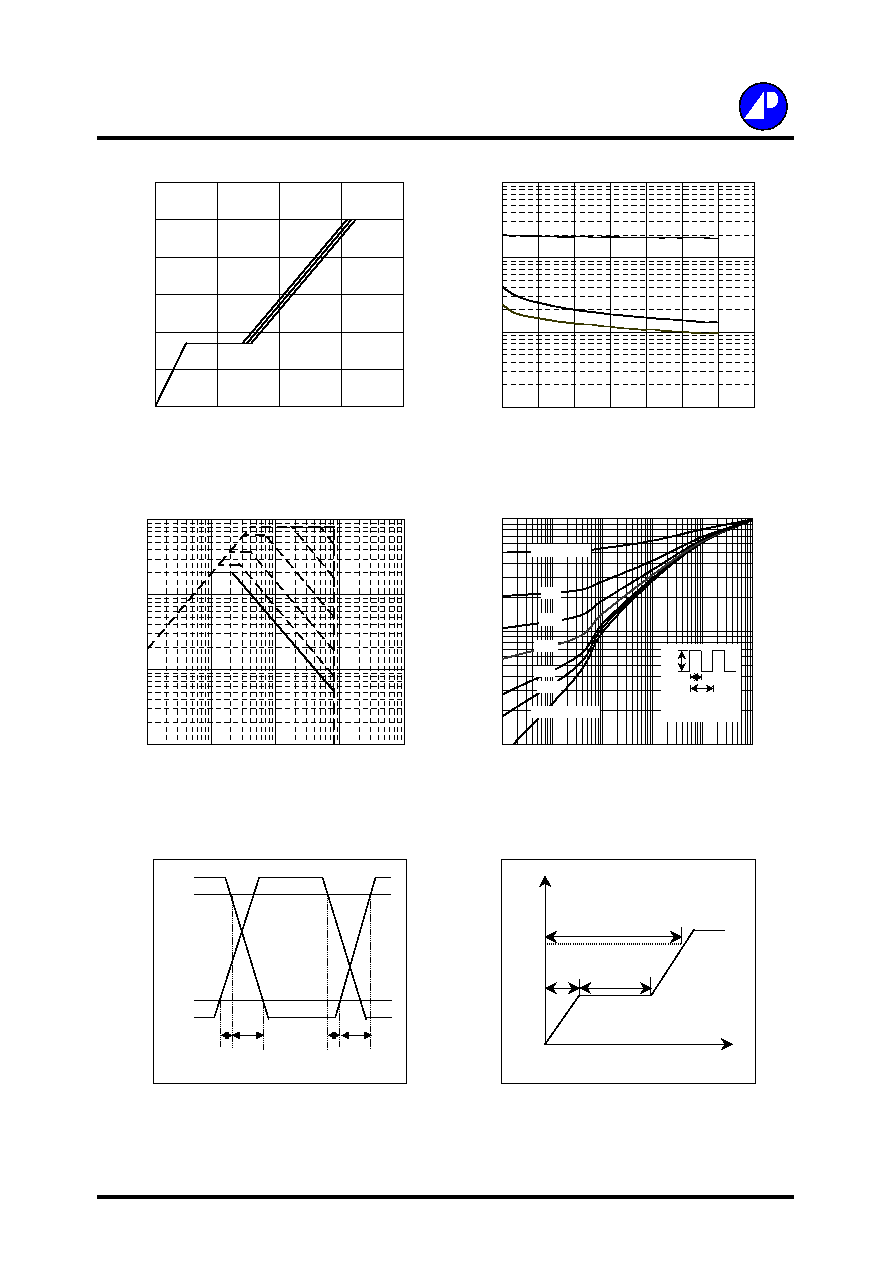Search datasheet (1.687.043 components) Search fieldPart namePart descriptionAbout site Manufacturers list ChipFindIC search engine AllXrefCross-reference database### Datasheet: AP9980J (Advanced Power Electronics Corp.)

N-CHANNEL ENHANCEMENT MODE
Download:PDFZIPN-CHANNEL ENHANCEMENT MODE
Electronics Corp.
POWER MOSFET
Low Gate Charge
BV
DSS
80V
Single Drive Requirement
R
DS(ON)
45m
Fast Switching Performance
I
D
21.3A
Description
Absolute Maximum Ratings
Symbol
Units
V
DS
V
V
GS
V
I
D
@T
C
=25
A
I
D
@T
C
=100
A
I
DM
A
P
D
@T
C
=25
W
W/
T
STG
T
J
Symbol
Value
Units
Rthj-c
Thermal Resistance Junction-case
Max.
3.0
/W
Rthj-a
Thermal Resistance Junction-ambient
Max.
110
/W
Data and specifications subject to change without notice
Thermal Data
Parameter
Storage Temperature Range
Total Power Dissipation
41.7
-55 to 150
Operating Junction Temperature Range
-55 to 150
Linear Derating Factor
0.33
Continuous Drain Current, V
GS
@ 10V
13.4
Pulsed Drain Current
1
80
Gate-Source Voltage
�25
Continuous Drain Current, V
GS
@ 10V
21.3
Parameter
Rating
Drain-Source Voltage
80
200406041
AP9980H/J
The Advanced Power MOSFETs from APEC provide the
designer with the best combination of fast switching,
ruggedized device design, low on-resistance and cost-
effectiveness.
G
D
S
The TO-252 package is universally preferred for all commercial-
industrial surface mount applications and suited for low voltage
applications such as DC/DC converters. The through-hole version
(AP9980J) are available for low-profile applications.
G
D
S
TO-251(J)
G D
S
TO-252(H)Electrical Characteristics@T
j
=25
o
C(unless otherwise specified)
Symbol
Parameter
Test Conditions
Min.
Typ. Max. Units
BV
DSS
Drain-Source Breakdown Voltage
V
GS
=0V, I
D
=250uA
80
-
-
V
B
V
DSS
/T
j
Breakdown Voltage Temperature Coefficient
Reference to 25
, I
D
=1mA
-
0.07
-
V/
R
DS(ON)
Static Drain-Source On-Resistance
2
V
GS
=10V, I
D
=12A
-
-
45
m
V
GS
=4.5V, I
D
=8A
-
-
55
m
V
GS(th)
Gate Threshold Voltage
V
DS
=V
GS
, I
D
=250uA
1
-
3
V
g
fs
Forward Transconductance
V
DS
=10V, I
D
=12A
-
20
-
S
I
DSS
Drain-Source Leakage Current (T
j
=25
o
C)
V
DS
=80V, V
GS
=0V
-
-
10
uA
Drain-Source Leakage Current (T
j
=150
o
C)
V
DS
=64V ,V
GS
=0V
-
-
100
uA
I
GSS
Gate-Source Leakage
V
GS
=�25V
-
-
nA
Q
g
Total Gate Charge
2
I
D
=12A
-
18
30
nC
Q
gs
Gate-Source Charge
V
DS
=64V
-
5
-
nC
Q
gd
Gate-Drain ("Miller") Charge
V
GS
=4.5V
-
11
-
nC
t
d(on)
Turn-on Delay Time
2
V
DS
=40V
-
11
-
ns
t
r
Rise Time
I
D
=12A
-
20
-
ns
t
d(off)
Turn-off Delay Time
R
G
=3.3
,
V
GS
=10V
-
29
-
ns
t
f
Fall Time
R
D
=3.3
-
30
-
ns
C
iss
Input Capacitance
V
GS
=0V
-
1810 2900
pF
C
oss
Output Capacitance
V
DS
=25V
-
135
-
pF
C
Reverse Transfer Capacitance
f=1.0MHz
-
96
-
pF
R
g
Gate Resistance
f=1.0MHz
-
1.6
-
Source-Drain Diode
Symbol
Parameter
Test Conditions
Min.
Typ. Max. Units
V
SD
Forward On Voltage
2
I
S
=20A, V
GS
=0V
-
-
1.2
V
t
rr
Reverse Recovery Time
2
I
S
=12A,
V
GS
=0
V
,
-
57
-
ns
Q
rr
Reverse Recovery Charge
dI/dt=100A/�s
-
140
-
nC
Notes:
1.Pulse width limited by safe operating area.
2.Pulse width <300us , duty cycle <2%.
AP9980H/J
100AP9980H/J
Fig 1. Typical Output Characteristics
Fig 2. Typical Output Characteristics
t
rr
Q
rr
Fig 3. On-Resistance v.s. Gate Voltage
Fig 4. Normalized On-Resistance
v.s. Junction Temperature
Fig 5. Forward Characteristic of
Fig 6. Gate Threshold Voltage v.s.
Reverse Diode
Junction Temperature
0
10
20
30
40
50
60
0
3
6
9
12
15
18
V
DS
, Drain-to-Source Voltage (V)
I
D
, Drain
Cu
rre
n
t
(A)
T
C
=25
o
C
10V
6.0V
5.0V
4.5V
V
G
=3.0V
0
10
20
30
40
50
0
3
6
9
12
15
18
V
DS
, Drain-to-Source Voltage (V)
I
D
, Drain
Cu
rre
n
t
(A)
T
C
=150
o
C
10V
6.0V
5.0V
4.5V
V
G
=3.0V
38
42
46
50
54
3
5
7
9
11
V
GS
, Gate-to-Source Voltage (V)
R
DS(
ON)
(m

)
I
D
= 8 A
T
C
=25
o
C
0.4
0.6
0.8
1.0
1.2
1.4
1.6
1.8
2.0
2.2
-50
0
50
100
150
T
j
, Junction Temperature (
o
C)
N
o
rmalize
d
R
DS(
ON)
I
D
= 12 A
V
G
=10V
0
0.5
1
1.5
2
2.5
3
-50
0
50
100
150
T
j
, Junction Temperature (
o
C)
V
GS(
t
h)
(V
)
0
2
4
6
8
0
0.2
0.4
0.6
0.8
1
1.2
V
SD
, Source-to-Drain Voltage (V)
I
S
(A
)
T
j
=25
o
C
T
j
=150
o
CFig 7. Gate Charge Characteristics
Fig 8. Typical Capacitance Characteristics
Q
rr
Fig 9. Maximum Safe Operating Area
Fig 10. Effective Transient Thermal Impedance
Fig 11. Switching Time Waveform
Fig 12. Gate Charge Waveform
AP9980H/J
t
d(on)
t
r
t
d(off)
t
f
V
DS
V
GS
10%
90%
Q
V
G
4.5V
Q
GS
Q
GD
Q
G
Charge
0
2
4
6
8
10
12
0
10
20
30
40
Q
G
, Total Gate Charge (nC)
V
GS
, Gate
to S
o
u
r
c
e
Voltage
(V)
I
D
= 12 A
V
DS
= 4 0V
V
DS
= 50 V
V
DS
= 64 V
10
100
1000
10000
1
5
9
13
17
21
25
29
V
DS
, Drain-to-Source Voltage (V)
C (
p
F)
f=1.0MHz
C
iss
C
oss
C
0.01
0.1
1
0.00001
0.0001
0.001
0.01
0.1
1
t , Pulse Width (s)
N
o
rmalize
d
The
r
mal Re
sponse
(
R
thjc
)
P
DM
Duty factor = t/T
Peak T
j
= P
DM
x R
thjc
+ T
C
t
T
0.02
0.01
0.05
0.1
0.2
Duty factor=0.5
Single Pulse
0.1
1
10
100
0.1
1
10
100
1000
V
DS
, Drain-to-Source Voltage (V)
I
D
(A
)
10us
100us
1ms
10ms
100ms
DC
T
C
=25
o
C
Single Pulse© 2020 • ICSheet• Contact form• Main page